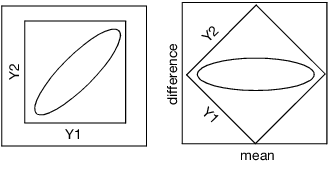Publication date: 08/13/2020

## Correlation of Responses

In most cases where the pair of measurements is taken from the same individual at different times, they are positively correlated. However, if they represent competing responses, the correlation can be negative.

Figure 19.7 shows how the positive correlation of the two responses becomes the small variance on the difference (the vertical axis). If the correlation is negative, the ellipse is oriented in the other direction and the variance of the rotated graph is large on the vertical axis.

Figure 19.7 Examples of Positive Correlation Before and After Rotation